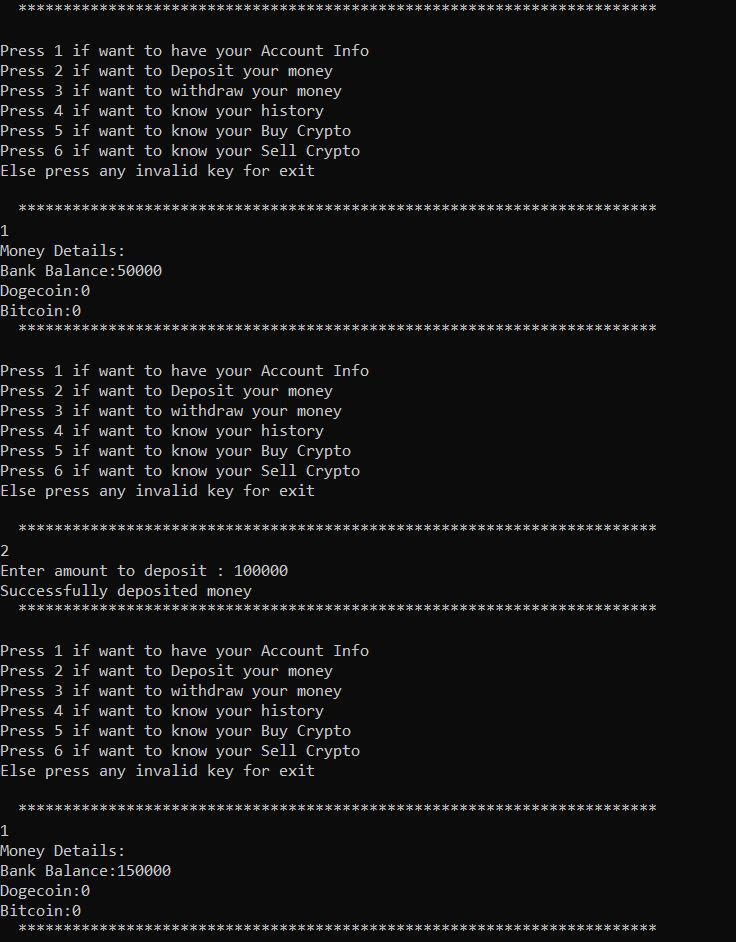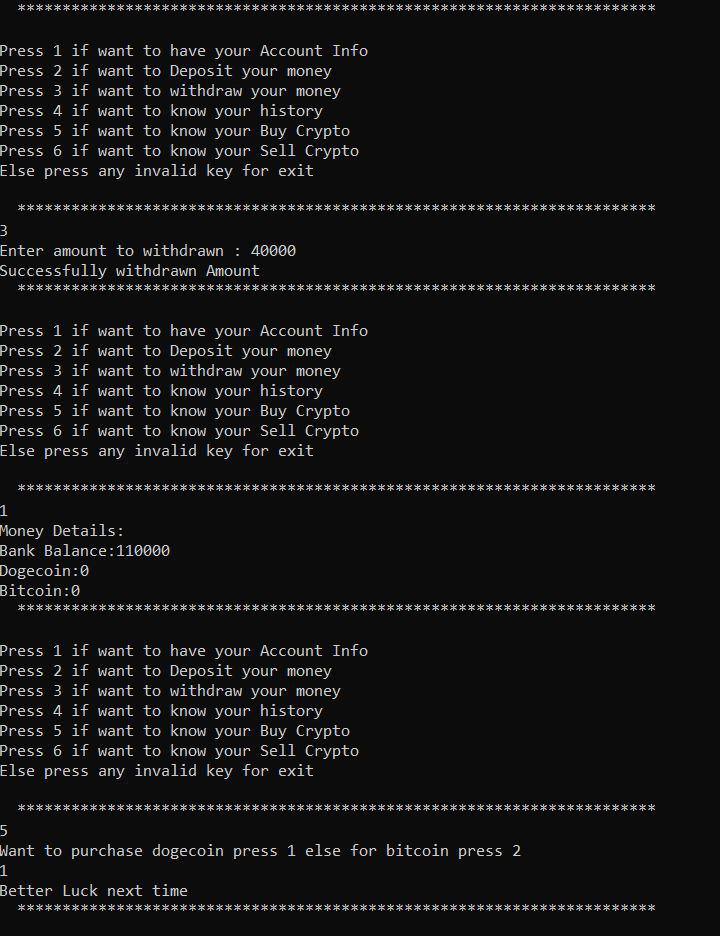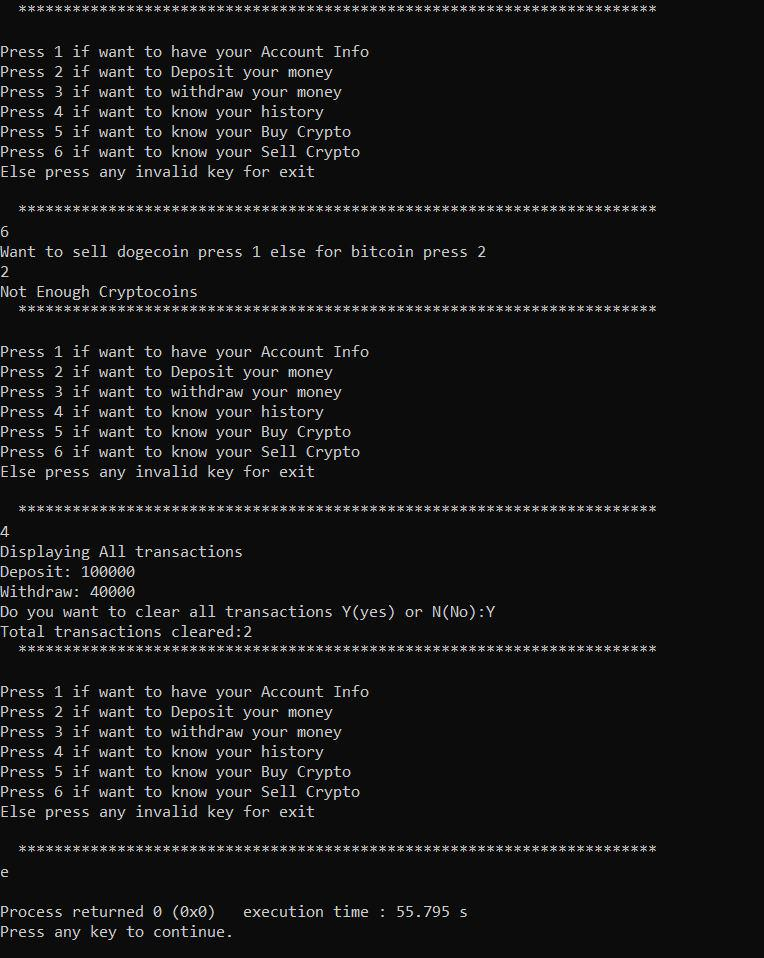Open in App
Not now

# C++ Program to Implement Trading Application

• Difficulty Level : Easy
• Last Updated : 26 Dec, 2022

Prerequisite:

Trading is a primary economic concept that involves buying and selling goods and services, along with compensation paid by a buyer to a seller. Trading applications in C++ can be a good example of understanding the basic concepts of C++. Also, It can act as a mini-projects for beginners, which can help them to enhance the concepts of C++ and also can build up their resume in the initial stage.

### Problem Statement:

Build a Program in C++ forTrading applications where the operations performed are given below:

1. Get account Information
2. Deposit Money
3. Withdraw Money
5. Sell Cryptocoins
6. Check Transactions

### Operations to be Performed

Trading includes knowledge about the profit loss and currencies. So the variables of the program will be as follows:

1.  Balance of user
2.  Profit Loss checker
3.  Bitcoins and Dogecoin
4.  Choice
5.  Withdraw amount
6.  Predict
7.  Total equity

Now about the Operations, we will be Performing:

#### 1. Get Account Information:

In this operation, we will Just print the information about the user account. i.e. all the variables we have to observe are profit-loss, currencies, amount, etc.

#### 2. Deposit Money:

In deposit, we can deposit money in our account.

#### 3. Withdraw Money:

In withdrawal, we can withdraw the money we have in our account.

We will buy crypto using this operation. We can buy either Bitcoin or Dogecoin depending on our needs.

#### 5. Sell Cryptocurrencies:

We will sell crypto and add the amount we get after selling to our account.

#### 6. Check Transactions:

In this Operation, we will print all the transactions performed till now.

## C++

 `// C++ Program to create` `// Trading application` `#include ` `using` `namespace` `std;`   `// Class created for binding` `// all account details together` `class` `Account {` `    ``// Basic Variables declared` `    ``int` `dogecoin, balance;` `    ``int` `bitcoin;` `    ``int` `deposit, withdraw;` `    ``int` `total_equity = 100;` `    ``int` `predict;` `    ``int` `dogecoin_value;` `    ``int` `bitcoin_value;` `  ``int` `crypto_invest;` `int` `crypto_return;`   `    ``// Vector declared` `    ``vector > transactions;`   `public``:` `    ``// Deposit Cash` `    ``bool` `Deposit(``int` `money)` `    ``{` `        ``deposit += money;` `        ``balance += money;` `        ``transactions.push_back({ ``"Deposit:"``, money });` `    ``}`   `    ``// Get account Details` `    ``void` `Get_account_information()` `    ``{`   `        ``cout << ``"Money Details:\n"``;` `        ``cout << ``"Bank Balance:"` `<< balance << endl;` `        ``cout << ``"Dogecoin:"` `<< dogecoin << endl;` `        ``cout << ``"Bitcoin:"` `<< bitcoin << endl;` `    ``}`   `    ``// Withdraw Cash` `    ``bool` `Withdraw(``int` `money)` `    ``{` `        ``if` `(money > balance) {` `            ``return` `false``;` `        ``}`   `        ``withdraw += money;` `        ``balance -= money;` `        ``transactions.push_back({ ``"Withdraw:"``, money });` `        ``return` `true``;` `    ``}`   `    ``// Buy Crypto function` `    ``bool` `buy_crypto()` `    ``{` `        ``int` `option;` `        ``cout << ``"Want to purchase dogecoin press 1 else "` `                ``"for bitcoin press 2\n"``;` `        ``cin >> option;`   `        ``// Checking equity` `        ``if` `(total_equity != 0) {` `            ``srand``(``time``(NULL));` `            ``int` `luck = ``rand``();`   `            ``// Checking if random number is divisible by` `            ``// 251 to check (random case for buying  crypto` `            ``// used)` `            ``if` `(luck % 251 == 0) {` `                ``if` `(option == 1) {` `                    ``dogecoin += 1;` `                    ``balance -= dogecoin_value;` `                    ``crypto_invest` `                        ``+= (dogecoin)*dogecoin_value;` `                ``}` `                ``else` `{` `                    ``bitcoin += 1;` `                    ``balance -= bitcoin_value;` `                    ``crypto_invest += bitcoin_value;` `                ``}` `            ``}` `            ``else` `{` `                ``return` `false``;` `            ``}` `        ``}` `        ``else` `{` `            ``return` `false``;` `        ``}`   `        ``return` `true``;` `    ``}`   `    ``// Selling crypto function` `    ``bool` `sell_crypto()` `    ``{` `        ``int` `option;` `        ``cout << ``"Want to sell dogecoin press 1 else for "` `                ``"bitcoin press 2\n"``;` `        ``cin >> option;`   `        ``if` `(option == 2) {` `            ``if` `(bitcoin == 0)` `                ``return` `false``;` `            ``crypto_return += bitcoin_value;` `            ``balance += bitcoin_value;` `            ``transactions.push_back(` `                ``{ ``"Bitcoin Sold:"``, bitcoin_value });` `            ``bitcoin -= 1;` `        ``}` `        ``else` `{` `            ``if` `(dogecoin == 0)` `                ``return` `false``;` `            ``crypto_return += dogecoin_value;` `            ``balance += dogecoin_value;` `            ``transactions.push_back(` `                ``{ ``"Dogecoin Sold:"``, dogecoin_value });` `            ``dogecoin -= 1;` `        ``}`   `        ``return` `true``;` `    ``}`   `    ``// Checking All Transactions` `    ``void` `History()` `    ``{` `        ``cout << ``"Displaying All transactions\n"``;` `        ``for` `(``auto` `it : transactions) {` `            ``cout << it.first << ``" "` `<< it.second << endl;` `        ``}`   `        ``char` `temp;` `        ``cout << ``"Do you want to clear all transactions:"``;` `        ``cin >> temp;`   `        ``int` `no = transactions.size();`   `        ``// Clearing All transactions` `        ``if` `(temp == ``'Y'``) {` `            ``transactions.clear();` `            ``cout << ``"Total transactions cleared:"` `<< no` `                 ``<< endl;` `        ``}` `        ``else` `{` `            ``cout << ``"Total transaction:"` `<< no << endl;` `        ``}` `    ``}`   `    ``Account()` `    ``{` `        ``crypto_invest = 0;` `        ``crypto_return = 0;` `        ``total_equity = 100;`   `        ``balance = 50000;` `        ``dogecoin = 0;` `        ``bitcoin = 0;` `        ``withdraw = 0;` `        ``deposit = 0;` `        ``dogecoin_value = 100;` `        ``bitcoin_value = 500;` `    ``}` `};`   `int` `main()` `{` `    ``Account person;` `    ``int` `amount, choice;` `    ``bool` `check;` `    ``while` `(1) {`   `        ``cout << ``"  "` `                ``"******************************************"` `                ``"*****************************  \n"``;`   `        ``cout << endl;` `        ``cout << ``"Press 1 if want to have your Account Info "` `             ``<< endl;` `        ``cout << ``"Press 2 if want to Deposit your money "` `             ``<< endl;` `        ``cout << ``"Press 3 if want to withdraw your money "` `             ``<< endl;` `        ``cout << ``"Press 4 if want to know your history "` `             ``<< endl;` `        ``cout << ``"Press 5 if want to know your Buy Crypto "` `             ``<< endl;` `        ``cout << ``"Press 6 if want to know your Sell Crypto "` `             ``<< endl;` `        ``cout << ``"Else press any invalid key for exit \n"` `             ``<< endl;`   `        ``cout << ``"  "` `                ``"******************************************"` `                ``"*****************************  \n"``;`   `        ``cin >> choice;` `        ``int` `ans;`   `        ``switch` `(choice) {` `        ``case` `1:` `            ``person.Get_account_information();` `            ``break``;`   `        ``case` `2:` `            ``cout << ``"Enter amount to deposit : "``;` `            ``cin >> amount;` `            ``ans = person.Deposit(amount);` `            ``if` `(ans)` `                ``cout << ``"Successfully deposited money"` `                     ``<< endl;` `            ``else` `                ``cout << ``"Failed\n"``;`   `            ``break``;`   `        ``case` `3:` `            ``cout << ``"Enter amount to withdrawn : "``;` `            ``cin >> amount;` `            ``person.Withdraw(amount);`   `            ``if` `(ans)` `                ``cout << ``"Successfully withdrawn Amount"` `                     ``<< endl;` `            ``else` `                ``cout << ``"Not Enough Balance\n"``;`   `            ``break``;`   `        ``case` `4:` `            ``person.History();` `            ``break``;`   `        ``case` `5:` `            ``ans = person.buy_crypto();` `            ``if` `(ans)` `                ``cout << ``"Successful Transaction"` `<< endl;` `            ``else` `                ``cout << ``"Better Luck next time\n"``;` `            ``break``;`   `        ``case` `6:` `            ``ans = person.sell_crypto();` `            ``if` `(ans)` `                ``cout << ``"Successful Transaction"` `<< endl;` `            ``else` `                ``cout << ``"Not Enough Cryptocoins\n"``;` `            ``break``;`   `        ``default``:` `            ``exit``(0);` `            ``break``;` `        ``}` `    ``}` `}`

Output

```  ***********************************************************************

Press 1 if want to have your Account Info
Press 2 if want to Deposit your money
Press 3 if want to withdraw your money
Press 4 if want to know your history
Press 6 if want to know your Sell Crypto
Else press any invalid key for exit

***********************************************************************
```

Output: